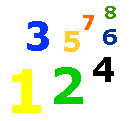# 3-58 As Time Goes By!

### Lesson List

Learn to tell how much time has passed (elapsed) in minutes.

Look at the two clocks below.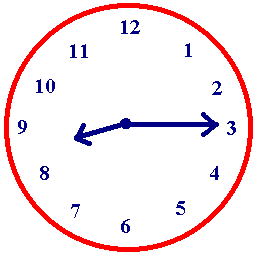The clock above reads 8:15.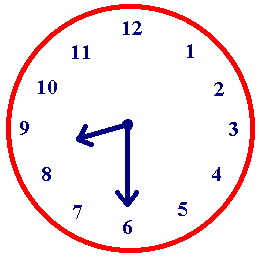The clock above reads 8:30.

The difference between 8:15 and 8:30 is 15 minutes.

From 8:15 to 8:30 is 15 minutes because 30 -15 = 15.  Because the hours are the same, you only need to subtract the minutes to find the difference.

Look at the two clocks below.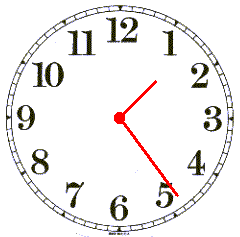The clock above reads 1:24.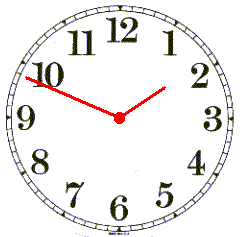The clock above reads 1:49.

To find the difference (the minutes that passed) between 1:49 and 1:24 you need to subtract the minutes.

49 - 24 = 25.

There is a 25 minute difference between 1:24 and 1:49.

When the hours are different, you need to subtract and add.  Look at the clocks below.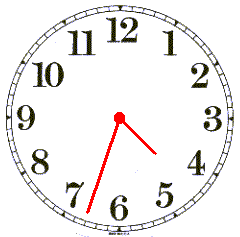The clock above reads 4:33.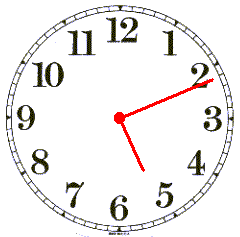The clock above reads 5:11.

You will need to do this problem in two steps.

First, find how many minutes it is until 5:00.  To do this, subtract 33 minutes from 60 minutes.

60 - 33 = 27.

Next, add the 11 minutes past 5:00.  This is the difference between 5:00 and 5:11.

27 + 11 = 38

The difference between 4:33 and 5:11 is 38 minutes.

Complete the problems below.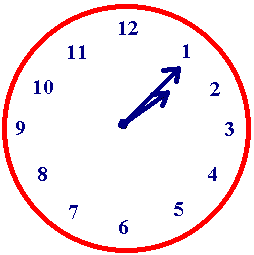The time is 1:05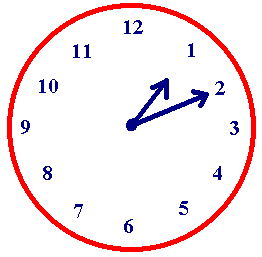The time is 1:10.The difference between 1:05 and 1:10 is minutes.The time is 1:10.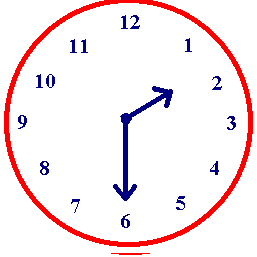The time is 1:30The difference between 1:10 and 1:30 is minutes.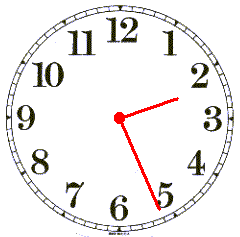The time is 2:26.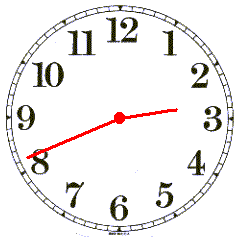The time is 2:41.The difference between 2:26 and 2:41 is minutes.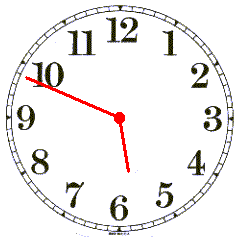The difference between the clocks above is minutes.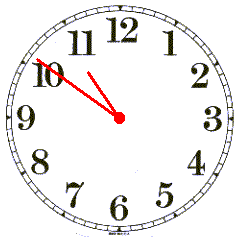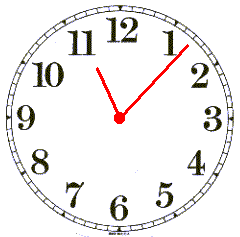The difference between the clocks above is minutes.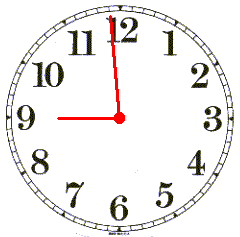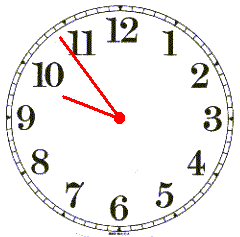The difference between the clocks above is minutes.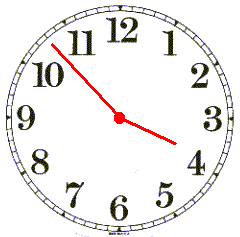The difference between the clocks above is minutes.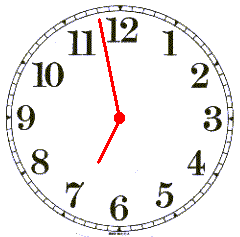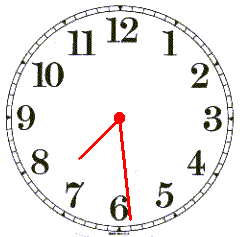The difference between the clocks above is minutes.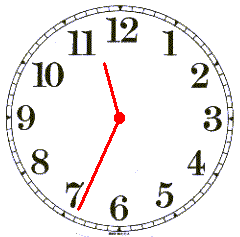The difference between the clocks above is minutes.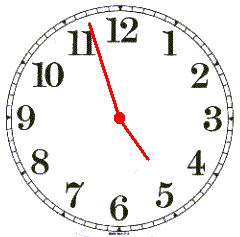The difference between the clocks above is 1 hour and minutes.